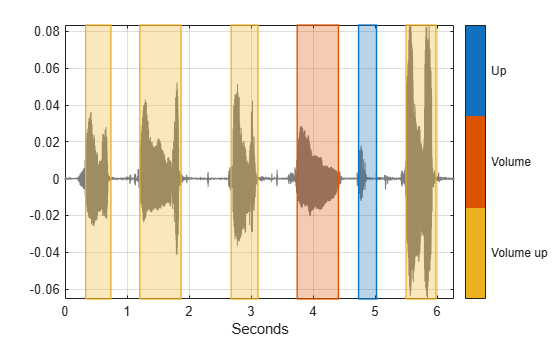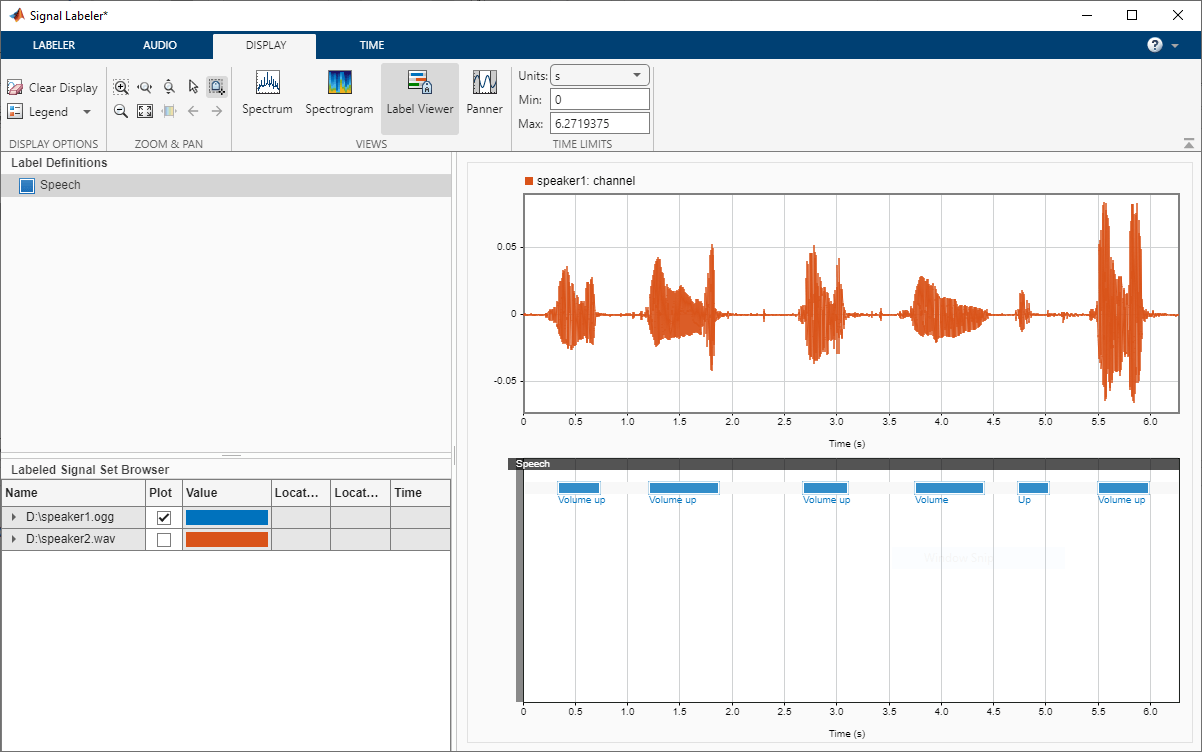# Import Audacity Labels to Signal Labeler

This example shows how to import labels created in Audacity™ into Signal Labeler.

### Read Labels in MATLAB

You have an audio file consisting of a human voice uttering "Volume up" several times.

Load the audio file to the MATLAB® workspace.

```audioFile = "speaker1.ogg"; [x,fs] = audioread(audioFile); sound(x,fs)```

You label the file in Audacity  and export the labels to `speaker.txt`.

Read the labels using `readtable`. The labels consist of three columns corresponding to the speech utterances along with their respective start and end times (in seconds).

```labelFile = "speaker1.txt"; roiTable = readtable(labelFile,Delimiter="tab"); roiTable.Properties.VariableNames = ["StartTime","EndTime","Value"]```
```roiTable=6×3 table StartTime EndTime Value _________ _______ _____________ 0.33273 0.74033 {'Volume up'} 1.2062 1.8716 {'Volume up'} 2.6785 3.111 {'Volume up'} 3.7432 4.4087 {'Volume' } 4.7331 5.0243 {'Up' } 5.4984 5.9809 {'Volume up'} ```

In order to gain insight into the labels, you plot the audio signal along with a mask corresponding to labeled regions of speech.

Convert the signal regions of interest to a binary mask.

```mask=signalMask(table(roiTable{:,1:2},categorical(roiTable{:,3})),SampleRate=fs); plotsigroi(mask, x, true);```### Convert Labels to a Labeled Signal Set

Next, convert the label data to a `labeledSignalSet` that can be imported to Signal Labeler.

First, define the label type. Specify `"roi"` (Region of interest) for the label type, and `"string"` for the label datatype.

```labelName = "Speech"; lblDef = signalLabelDefinition(string(labelName),... LabelType="roi",... LabelDataType="string");```

Next, create a `labeledSignalSet` pointing to the labeled audio file. Add the label definition to the labeled signal set.

`lss = labeledSignalSet(audioDatastore(audioFile),lblDef);`

Set the label values.

```roiLimits = [roiTable.StartTime roiTable.EndTime]; setLabelValue(lss,1,labelName,roiLimits,string(roiTable.Value));```

### Load Labels in Signal Labeler

You are now ready to read these labels into Signal Labeler.

1) Open `signalLabeler`

2) Click Import, then `From Workspace`, and import `lss`.

The audio signal is now available to you along with its labels.

### Import a Labeled Dataset

The helper function `importLabels` creates a labeled signal set corresponding to labels for multiple audio files. In this example, you work with two audio files with labels stored in text files.

Call `importLabels` to create a signal data set for multiple annotated files. Specify the label name as `"Speech"`.

`lss = importLabels("Speech");`

You can now load `lss` in `SignalLabeler` by following the same steps from the previous section.```function lss = importLabels(labelName) % IMPORTLABELS Import labels from multiple audio file % % The function assumes the labels are stored in *.txt files % The function assumes the label file name is identical to the % audio file name. labelFiles = dir("*.txt"); labelFiles = {labelFiles.name}; numFiles = length(labelFiles); % Create an audio datastore pointing to all audio files in the current % folder ads = audioDatastore(pwd); lss = labeledSignalSet(ads); lblDef = signalLabelDefinition(string(labelName),... LabelType="roi",... LabelDataType="string"); addLabelDefinitions(lss,lblDef) for index0=1:numFiles filename = labelFiles{index0}; roiTable = readtable(filename,Delimiter="tab"); roiTable.Properties.VariableNames = ["StartTime","EndTime","Value"]; roiLimits = [roiTable.StartTime roiTable.EndTime]; setLabelValue(lss,index0,labelName,roiLimits,roiTable.Value); end end```